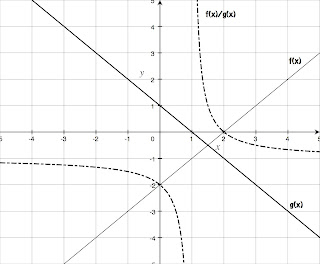## Wednesday, February 21, 2007

### Rational Functions

This post is in response to Lsquared's question in the previous post.

Here is the basic outline for my rational functions unit:

1.Introduce the shape of the hyperbola by giving students two linear functions, and having them divide the y-values for a bunch of different x-values. This shows that the x-intercept occurs when the numerator line has an x-intercept, and the vertical asymptote occurs when the denominator line has an x-intercept. My students also need some understanding about what happens when you divide a constant by a smaller and smaller number, so we do some numerical work with tables here, as well as conceptual understanding of what dividing by a smaller and smaller fraction means.

2. After this, we begin some basic feature analysis of simple hyperbolas. Students should be able to look at a graph and/or equation of a simple hyperbola and be able to quickly determine the domain, range, vertical asymptote, and intercepts. We practice matching graphs to equations and generating graphs by hand.

3. At this point, I want them to start thinking about end behavior, so we bring back polynomial division. We divide the numerator by the denominator, and then talk about what happens as "x gets really big". This is good scaffolding for limits. Last year, I held students accountable for determining all sorts of end-behavior by this method - not just horizontal asymptotes. I'm not sure if this is too much for them at this level or not. They definitely struggled with it last year.

4. Last year, I had students practice with more complicated hyperbolas, that had multiple vertical asymptotes (but no holes yet). I think I will cut this for this year, mainly to save time.

5. Now we look at what happens when you reduce a rational function, and discuss functions that are the same at all but one point, like f(x) = x^2/x and g(x) = x. We discuss holes in graphs, and when they occur. Students have trouble determining when a value for x causes a hole, a vertical asymptote, or an x-intercept, so we spend some time really focusing in on those concepts.

6. After this, I spend a couple of lessons just having them practice analyzing and graphing rational functions, using all that they've learned so far.

7. When I think students have a clear picture of what is happening, we move in to operations. I start them with multiplying and dividing rational functions, as that is much easier to do.

8. In the following lesson, we practice factoring and finding the LCD, so we can add and subtract rational functions.

9. Finally, we have a couple of lessons where we work on solving rational equations. I teach them multiplying by the LCD and cross-multiplication as two main strategies to use. Students had difficulty last year with understanding extraneous solutions, so I'm going to need to think that through better this time around.

That's it. We have a full period review, and then the unit test.

This may change, as I haven't yet begun really planning the unit for this year yet, but I think I will be sticking with the same basic outline. Lsquared - I am definitely interested in hearing how you teach rationals. Anything I can steal?